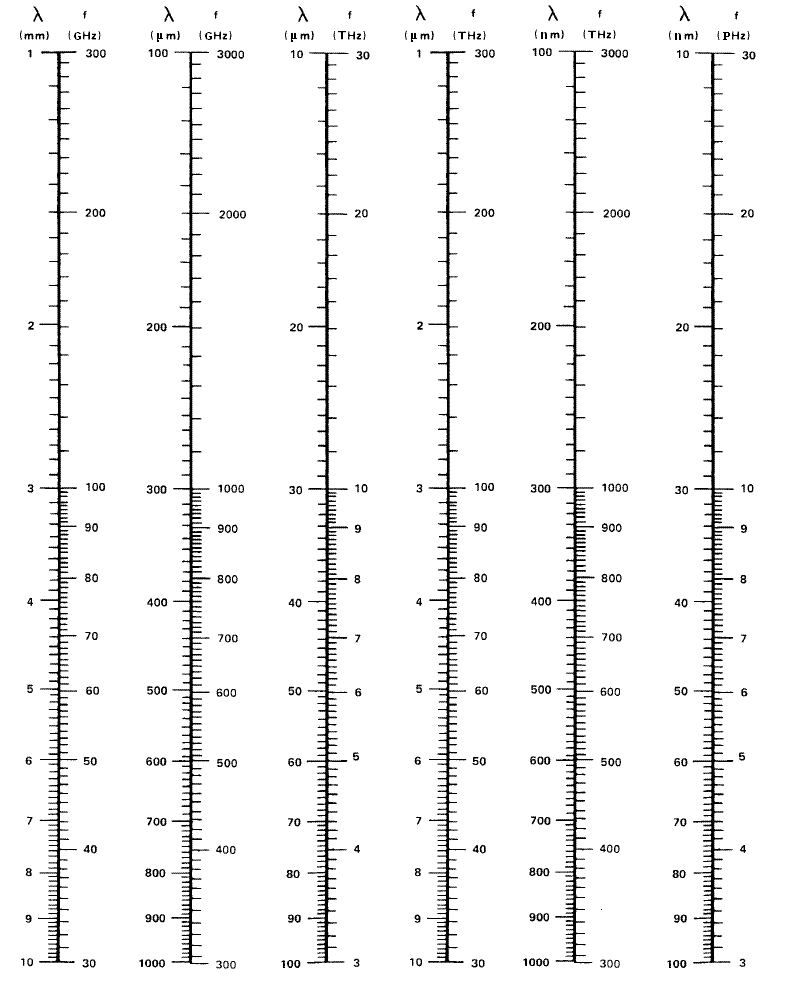BRIGHAM YOUNG UNIVERSITY
 Search BYUContact   |   Help[expand all...]
[COLLAPSE ALL...]# Frequency to Wavelength to Energy Calculator

## Frequency / Wavelength / Energy Calculator

To convert wavelength to frequency enter the wavelength in microns (μm) and press "Calculate f and E". The corresponding frequency will be in the "frequency" field in GHz.

OR enter the frequency in gigahertz (GHz) and press "Calculate λ and E" to convert to wavelength.
Wavelength will be in μm.

OR enter the energy in eV and click "Calculate λ and F" and the values will appear in the corresponding fields.

 Wavelength: (λ) [μm] Frequency: (f) [GHz] Energy: (E) [eV] Light type:
_______________________________________________________________________________________________________________

This chart shows wavelength in meters and frequency in hertz. By looking on the chart you may convert from wavelength to frequency and frequency to wavelength.
Frequency and wavelength of light are related to one another through the speed at which light travels.

Equation: f * λ = c
Equation: E = hc/λ

where:
f = frequency in Hertz (Hz = 1/sec)
λ = wavelength in meters (m)
c = the speed of light (299792458 m/s)
E = energy in electron Volts (eV)
h = Plank's constant (6.626068 × 10-34 m2kg/s)

_______________________________________________________________________________________________________________

# Conversion Chart

Original Nomograph - Maury Microwave Corp. '97

Nomograph conversion from λ = 1 mm to 10 nmNomograph conversion from λ = 1000 m to 1 cmRemember:

Mega-Hertz = MHz = 106 Hz
Giga-Hertz = GHz = 109 Hz
Tera-Hertz = THz = 1012 Hz
Peta-Hertz = PHz = 1015 Hz

milli-meters = mm = 10-3 m
microns = μm = 10-6 m
nano-meters = nm = 10-9 mMaintained by ECEn IMMERSE Web Team.